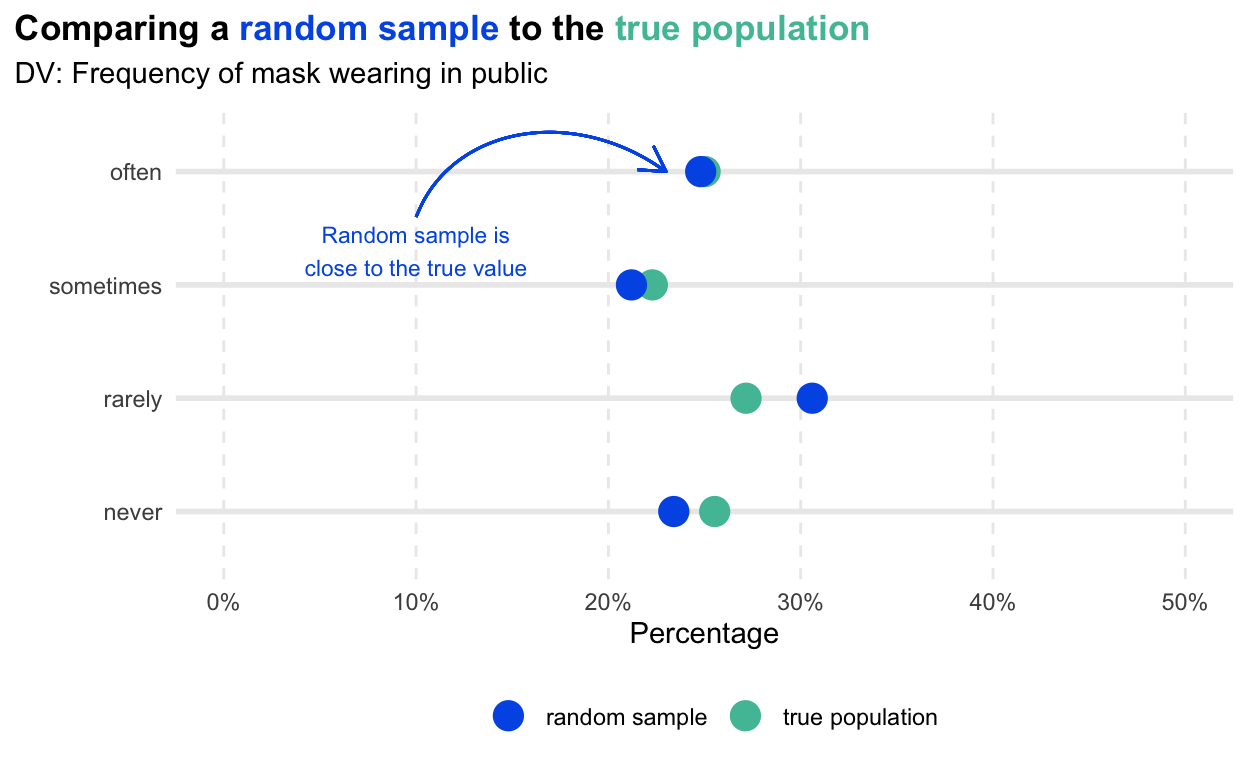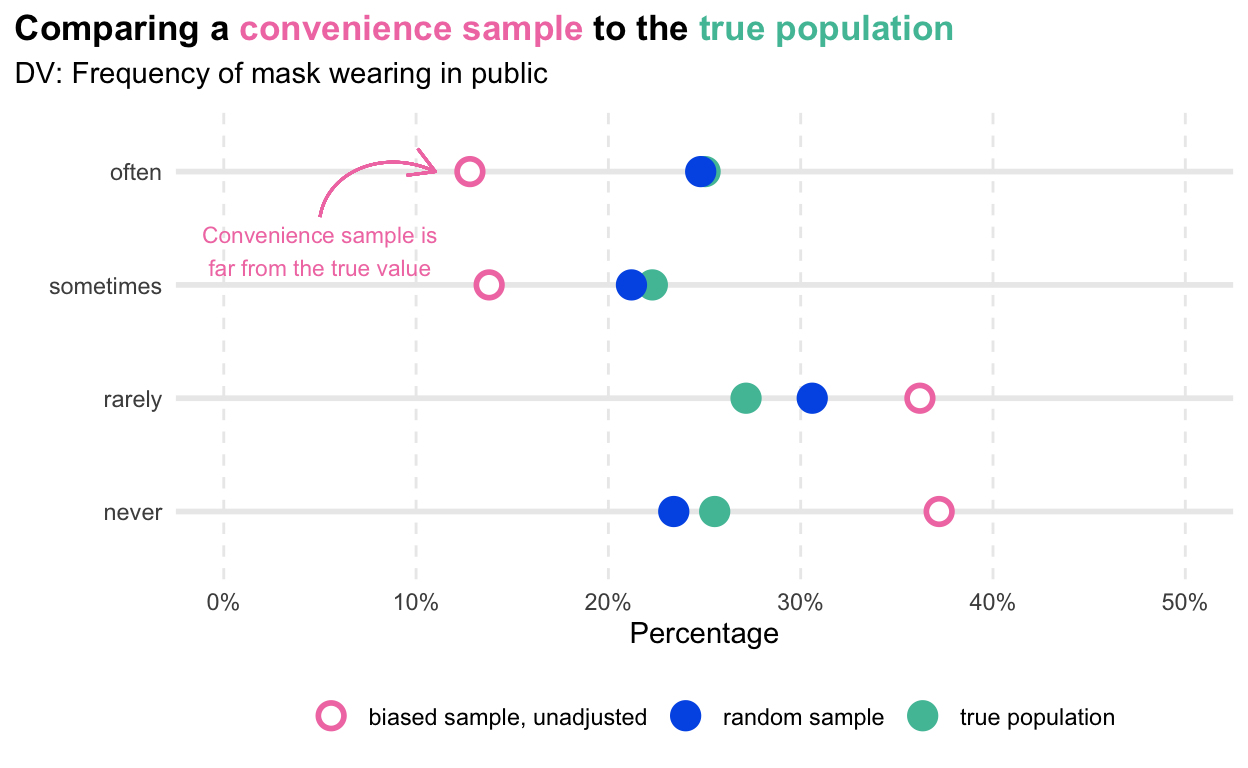# Mister P helps us understand vaccine hesitancy

In this post we imagine a fictional country called Dansia to illustrate how multilevel regression and post-stratification can help us to make sense of data from non-representative surveys.

true
12-08-2020

Previously I wrote about our efforts to monitor vaccine hesitancy through askNivi country polls. In our initial round in Kenya, we estimated that the probability is greater than 95% that at least 70% of people would be likely to get vaccinated if a vaccine were available for free today.

Skeptical readers might be thinking, “This is based on data from 486 people who responded to ads on Facebook. How is this representative of the country?” 🤔 Good question!

# Sampling 101

The gold standard approach for estimating characteristics of some population has long been probability-based representative sampling where every person in the target population has a chance of being invited to participate. In the global health world, the best examples come from the Demographic and Health Surveys (DHS) Program. DHS surveys involve between 5,000 and 30,000 households in a country and are typically conducted every 5 years. They cover dozens of topics and produce nationally-representative estimates through multistage cluster sampling. But there’s a catch: DHS surveys take 18 to 20 months (and a lot of money) to design, conduct door-to-door, and analyze. Despite their many strengths, DHS surveys are not an option when you need answers quickly.

A popular alternative is to contact a random sample of phone numbers and conduct the survey via SMS or phone. For instance, researchers might partner with a telecommunications company to get a list of all phone subscribers and randomly select numbers from this list, also known as a sampling frame. Another approach is called random digit dialing, where researchers randomly generate a list of phone numbers and try each number on the list (some will work, some won’t). While low cost and quick, poor coverage and high non-response can make these methods no better than non-representative convenience samples.1

Falling response rates in traditional public opinion polling, along with the growing ease of collecting data online, have led researchers to develop techniques for making convenience samples more representative (Wang et al. 2015). Before exploring one such approach, it will help to build some intuition about why just relying on convenience samples without any adjustment is usually a bad idea.

# An Inconvenient Truth

Imagine a country with 1 million residents. Let’s call it Dansia. Since we’re imagining things, let’s pretend that the good people of Dansia conducted a census last week of all 1 million residents and the data are ready to share. As luck would have it, the census included a question about our key dependent variable—frequency of mask wearing in public among people ages 15 to 64. This means we can calculate the true frequency in the population. And since we know the “real” answer, we can compare how different sampling approaches recover the true value.

  library(tidyverse)
library(ggtext)
library(gtsummary)
library(gt)

# get the population data and custom ggplot function

pop_df %>%
dplyr::select(-ageCat) %>%
mutate(gender = factor(gender,
levels=c("male", "female", "non-binary"),
labels=c("Male", "Female", "Non-binary"))) %>%
tbl_summary(by=gender,
label = c(age ~ "Mean age (SD)",
statistic = list(all_continuous() ~ "{mean} ({sd})",
all_categorical() ~ "{p}%")
) %>%
bold_labels() %>%
modify_footnote(update = everything() ~ NA) %>%
as_gt() %>%
tab_header(title="Population of Dansia, Ages 15 to 64") %>%
gt::tab_style(
style = list(gt::cell_fill(color = "#4FBFA5"),
gt::cell_text(color = "white",
weight="bold")),
locations = gt::cells_body(columns = vars(stat_0),
rows = 3:6)
)

Population of Dansia, Ages 15 to 64
Characteristic Overall, N = 681,732 Male, N = 323,970 Female, N = 323,758 Non-binary, N = 34,004
Mean age (SD) 39 (14) 39 (14) 39 (14) 39 (14)
never 26% 33% 18% 26%
rarely 27% 33% 21% 27%
sometimes 22% 19% 26% 22%
often 25% 16% 35% 25%

Remember, we only know this distribution of mask wearing in the population because we’re pretending we have timely census results. This would not happen in reality (and the census is not going to ask about mask wearing). In reality, we’d need to infer information about the population from a sample of people we contact. So let’s do that. First we’ll draw a random sample, followed by a convenience sample.

## Random Sample

The gold standard approach to sampling from this population would be to draw a random sample from a sampling frame. Let’s assume we could do this from a phone book and contacted 500 randomly selected people. Let’s also assume that we achieved 100% coverage and 0% non-response!

  library(tidyverse)
library(ggtext)

# get the population data and custom ggplot function

# draw random sample
set.seed(12345)
sample_rand <- pop_df %>%
sample_n(500)

# calculate response frequency in the population
overall_pop <- pop_df %>%
group_by(dv) %>%
count() %>%
ungroup() %>%
mutate(p = n/sum(n)) %>%
mutate(source = "true population") %>%
dplyr::select(source, dv, p)

# calculate response frequency in the random sample
overall_sample_rand <- sample_rand %>%
group_by(dv) %>%
count() %>%
ungroup() %>%
mutate(p = n/sum(n)) %>%
mutate(source = "random sample") %>%
dplyr::select(source, dv, p)

# combine and plot (uses custom ggplot function that comes with data)
overall_pop %>%
bind_rows(overall_sample_rand) %>%
mrp_plot(., plotTitle = "Comparing a <span style = 'color:#005BE7;'>random sample</span> to the <span style = 'color:#4FBFA5;'>true population</span>",
annLabel = "Random sample is\nclose to the true value",
ann_x = 3.3, ann_y = 0.10, annColor = "#005BE7",
arrow_x_start = 3.6, arrow_y_start = 0.10,
arrow_x_end = 4, arrow_y_end = 0.23, arrowColor = "#005BE7",
fills = c("#005BE7", "#4FBFA5"), colors = c("#005BE7", "#4FBFA5"))Figure 1: A random sample gets pretty close!

As you can see in Figure 1, our random sample gets pretty close to the true population values. Of course this is just one possible sample of 500 people from Dansia, but it illustrates the point that random samples are usually great (well, at least when coverage and response rates are high).

## Convenience Sample

Now let’s pull together a convenience sample and see how it compares to the population. “Convenience” means that we take who we get. Suppose we posted an advertisement online for people ages 15 to 64 to complete our survey. Some people in this age range would see the ad, most would not (poor coverage). And as it goes with ads, some people who see it will click, but most will not (high non-response).

# draw a convenience sample that is mostly young men
# ----------------------------------------------------
# 50 older men
sample_obs_m1 <- pop_df %>%
filter(gender=="male") %>%
filter(age>35) %>%
sample_n(50)

# 400 younger men
sample_obs_m2 <- pop_df %>%
filter(gender=="male") %>%
filter(age<=35) %>%
sample_n(400)

# 45 women
sample_obs_f <- pop_df %>%
filter(gender=="female") %>%
sample_n(45)

# 5 individuals who identified as non-binary
sample_obs_n <- pop_df %>%
filter(gender=="non-binary") %>%
sample_n(5)

# combine to make a sample of 500
sample_obs <- sample_obs_f %>%
bind_rows(sample_obs_m1) %>%
bind_rows(sample_obs_m2) %>%
bind_rows(sample_obs_n)

tbl_pop <- pop_df %>%
dplyr::select(-ageCat, -dv) %>%
mutate(gender = factor(gender,
levels=c("male", "female", "non-binary"),
labels=c("Male", "Female", "Non-binary"))) %>%
tbl_summary(label = c(age ~ "Mean age (SD)",
gender ~ "Gender"),
statistic = list(all_continuous() ~ "{mean} ({sd})",
all_categorical() ~ "{p}%")
) %>%
bold_labels() %>%
modify_footnote(update = everything() ~ NA)

tbl_rand <- sample_rand %>%
dplyr::select(-ageCat, -dv) %>%
mutate(gender = factor(gender,
levels=c("male", "female", "non-binary"),
labels=c("Male", "Female", "Non-binary"))) %>%
tbl_summary(label = c(age ~ "Mean age (SD)",
gender ~ "Gender"),
statistic = list(all_continuous() ~ "{mean} ({sd})",
all_categorical() ~ "{p}%")
) %>%
bold_labels() %>%
modify_footnote(update = everything() ~ NA)

tbl_obs <- sample_obs %>%
dplyr::select(-ageCat, -dv) %>%
mutate(gender = factor(gender,
levels=c("male", "female", "non-binary"),
labels=c("Male", "Female", "Non-binary"))) %>%
tbl_summary(label = c(age ~ "Mean age (SD)",
gender ~ "Gender"),
statistic = list(all_continuous() ~ "{mean} ({sd})",
all_categorical() ~ "{p}%")
) %>%
bold_labels() %>%
modify_footnote(update = everything() ~ NA)

tbl_merge(list(tbl_pop, tbl_rand, tbl_obs),
tab_spanner = c("**Population**", "**Random Sample**",
"**Convenience Sample**"))

Characteristic Population Random Sample Convenience Sample
N = 681,732 N = 500 N = 500
Gender
Male 48% 49% 90%
Female 47% 48% 9.0%
Non-binary 5.0% 3.4% 1.0%
Mean age (SD) 39 (14) 37 (14) 29 (11)

Our imaginary ad must have appealed more to younger, male audiences. Whereas males make up about half of the population and random sample, they are 90% of the convenience sample!

Now let’s estimate mask wearing in Dansia from this convenience sample that we know is younger and more male on average than the population.

# calculate response frequency in the random sample
overall_sample_obs <- sample_obs %>%
group_by(dv) %>%
count() %>%
ungroup() %>%
mutate(p = n/sum(n)) %>%
mutate(source = "biased sample, unadjusted") %>%
dplyr::select(source, dv, p)

# combine and plot
overall_pop %>%
bind_rows(overall_sample_rand) %>%
bind_rows(overall_sample_obs) %>%
mrp_plot(., plotTitle = "Comparing a <span style = 'color:#F17DB1;'>convenience sample</span> to the <span style = 'color:#4FBFA5;'>true population</span>",
annLabel = "Convenience sample is\nfar from the true value",
ann_x = 3.3, ann_y = 0.05, annColor = "#F17DB1",
arrow_x_start = 3.6, arrow_y_start = 0.05,
arrow_x_end = 4, arrow_y_end = 0.11, arrowColor = "#F17DB1",
fills = c("white", "#005BE7", "#4FBFA5"),
colors = c("#F17DB1", "#005BE7", "#4FBFA5"))Figure 2: Womp womp convenience sample.

Figure 2 is a bit depressing for #teamConvenience. The clue to why is in the first table (remember our fake census?): men in the population—particularly young men—endorsed mask wearing less often than women. Since our convenience sample skews male and younger, these anti-mask views are over-represented in our dataset. If we stopped here, we’d come to the wrong conclusion about the frequency of mask wearing in the population.

This, friends, is why convenience samples are hard to interpret when the goal is to make some inference about a target population.

# Multilevel Regression and Post-Stratification

But all is not lost. Take heart in this example from Wang et al. (2015) (pdf).

Andrew Gelman2 and colleagues ran daily voter polls on the Xbox gaming platform in the run up to the 2012 U.S. Presidential election. This was an opt-in poll. Every day, gamers were presented 3 to 5 questions, including the question, “If the election were held today, who would you vote for?”. The first time a person participated they were also asked to answer several demographic questions.